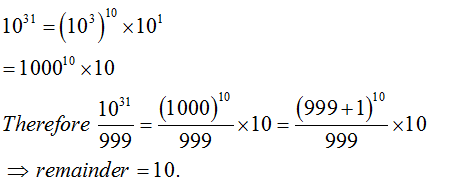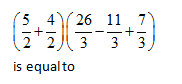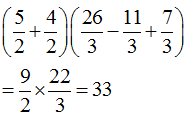• This is an assessment test.
• These tests focus on the basics of Maths and are meant to indicate your preparation level for the subject.
• Kindly take the tests in this series with a pre-defined schedule.

## Basic Maths: Test 38

Congratulations - you have completed Basic Maths: Test 38. You scored %%SCORE%% out of %%TOTAL%%. Your performance has been rated as %%RATING%%
 Question 1
Which one of the following does not divide$\left( {{5}^{2430}}-1 \right)$?
 A 24 B 124 C 624 D 3124
Question 1 Explanation:
When n is odd or even, ${{x}^{n}}-{{a}^{n}}$ is exactly divisible by x-a. \begin{align} & \left( {{5}^{2430}}-1={{25}^{1215}}-1,\,\,25-1\,\,\,i.e.\,\,is\,\,\,divisible\,\,by\,\,24 \right) \\ & {{5}^{2430}}-1={{\left( {{5}^{3}} \right)}^{810}}-1={{125}^{810}}-1, \\ & which\,\,\,\,is\,\,\,\,divisible\,\,by\,\,124. \\ & {{5}^{2430}}-1={{\left( {{5}^{5}} \right)}^{486}}-1, \\ & ={{\left( 3125 \right)}^{486}}-1\,\,which\,\,\,is\,\,\,divisible\,\,\,\,by\,3124. \\ \end{align}
 Question 2
When 1031 is divided by 999, the remainder is
 A 1 B 10 C 100 D 999
Question 2 Explanation:Question 3
Simplify: ${{\left( 0\overline{.2} \right)}^{2}}\left\{ \,3-11{{\left( 0.\overline{27} \right)}^{2}} \right\}$
 A $-\frac{32}{162}$ B $\frac{1}{297}$ C $\frac{76}{99}$ D $\frac{32}{297}$
Question 3 Explanation:
\begin{align} & {{\left( \frac{2}{9} \right)}^{2}}\left\{ 3-11{{\left( \frac{27}{99} \right)}^{2}} \right\} \\ & =\frac{4}{81}\left\{ 3-\frac{11\times 3\times 3}{11\times 11} \right\} \\ & =\frac{4}{81}\times \left\{ 3-\frac{9}{11} \right\} \\ & =\frac{4}{81}\times \left\{ \frac{24}{11} \right\} \\ & =\frac{4}{81}\times \frac{24}{11}=\frac{32}{297} \\ \end{align}
 Question 4
${{\left( 28 \right)}^{3}}+{{\left( 75 \right)}^{3}}-{{\left( 103 \right)}^{3}}+3\times 28\times 75\times 103$ is equal to:
 A 10000 B 0 C 30007 D 1
Question 4 Explanation:
\begin{align} & Let,\,\,28=\,\,a \\ & 75=\,b,\,-103=c \\ & When\,\,\left( a+b+c \right)=0 \\ & {{a}^{3}}+{{b}^{3}}+{{c}^{3}}-3abc=0 \\ & Here,\,\,a+b+c=\,28+75-103=0 \\ & Therefore\,\,{{(28)}^{3}}+{{\left( 75 \right)}^{3}}-{{\left( 103 \right)}^{3}}+3\times 28\times 75\times 103=0 \\ \end{align}
 Question 5A 33 B 19 C 37 D 36
Question 5 Explanation:Once you are finished, click the button below. Any items you have not completed will be marked incorrect.
There are 5 questions to complete.
 ← List →
Shaded items are complete.
 1 2 3 4 5 End